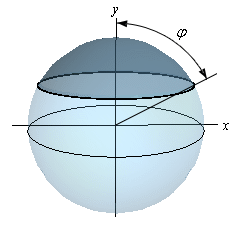Paul's Online Notes
Home / Calculus I / Applications of Integrals / More Volume Problems
Show Mobile Notice Show All Notes Hide All Notes
Mobile Notice
You appear to be on a device with a "narrow" screen width (i.e. you are probably on a mobile phone). Due to the nature of the mathematics on this site it is best views in landscape mode. If your device is not in landscape mode many of the equations will run off the side of your device (should be able to scroll to see them) and some of the menu items will be cut off due to the narrow screen width.
Assignment Problems Notice
Please do not email me to get solutions and/or answers to these problems. I will not give them out under any circumstances nor will I respond to any requests to do so. The intent of these problems is for instructors to use them for assignments and having solutions/answers easily available defeats that purpose.

If you are looking for some problems with solutions you can find some by clicking on the "Practice Problems" link above.

### Section 6.5 : More Volume Problems

1. Use the method of finding volume from this section to determine the volume of a sphere of radius $$r$$.
2. Find the volume of the solid whose base is the region bounded by $$x = 2 - {y^2}$$ and $$x = {y^2} - 2$$ and whose cross-sections are squares with the base perpendicular to the $$y$$-axis. See figure below to see a sketch of the cross-sections.3. Find the volume of the solid whose base is a disk of radius $$r$$ and whose cross-sections are rectangles whose height is half the length of the base and whose base is perpendicular to the $$x$$-axis. See figure below to see a sketch of the cross-sections (the positive $$x$$-axis and positive $$y$$-axis are shown in the sketch).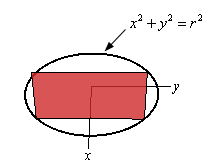4. Find the volume of the solid whose base is the region bounded by $$y = {x^2} - 1$$ and $$y = 3$$ and whose cross-sections are equilateral triangles with the base perpendicular to the $$y$$-axis. See figure below to see a sketch of the cross-sections.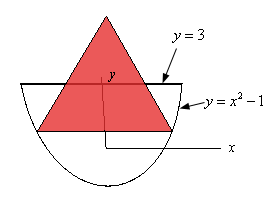5. Find the volume of the solid whose base is the region bounded by $$x = 2 - {y^2}$$ and $$x = {y^2} - 2$$ and whose cross-sections are the upper half of the circle centered on the $$y$$-axis. See figure below to see a sketch of the cross-sections.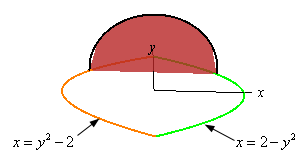6. Find the volume of a wedge cut out of a “cylinder” whose base is the region bounded by $$y = \cos \left( x \right)$$ and the $$x$$-axis between $$- \frac{\pi }{2} \le x \le \frac{\pi }{2}$$. The angle between the top and bottom of the wedge is $$\frac{\pi }{4}$$. See the figure below for a sketch of the “cylinder” and the wedge (the positive $$x$$-axis and positive $$y$$-axis are shown in the sketch).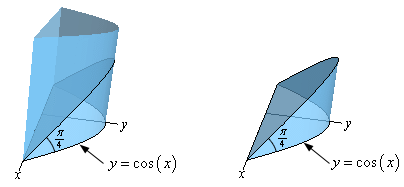7. For a sphere of radius $$r$$ find the volume of the cap which is defined by the angle $$\varphi$$ where $$\varphi$$ is the angle formed by the $$y$$-axis and the line from the origin to the bottom of the cap. See the figure below for an illustration of the angle $$\varphi$$.